# 链路Pn(m)优美性和序列性The Gracefulness and Sequences of Pn(m)

DOI: 10.12677/PM.2018.86097, PDF, HTML, XML, 下载: 573  浏览: 796

Abstract: The labelling of a graph G is injection g of the labels of vertices to a set of integers, and the labels of each edge e= uv are induced by the g(u) and g(v). In the paper, the k-gracefulness and sequence of graphs are given, and we also prove Pn(m) graph is k-graceful, sequential, and harmonious.

1. 引言及主要结果

$f:V\left(G\right)\to \left\{0,1,2,\cdots ,|E|+k-1\right\}$

${f}^{\prime }\left(uv\right)=|f\left(u\right)-f\left(v\right)|$

$E\left(G\right)\to \left\{k,k+1,\cdots ,k+|E|-1\right\}$

$\theta :V\left(G\right)\to \left\{0,1,2,\cdots ,|E|-1\right\}$

(如果G是树，则也包含|E|)使的由

${\theta }^{\prime }\left(uv\right)=\theta \left(u\right)+\theta \left(v\right)$

$E\left(G\right)\to \left\{c,c+1,\cdots ,c+|E|-1\right\}$

$V\left({P}_{n}\left({m}_{1},{m}_{2},\cdots ,{m}_{n-}{}_{2}\right)\right)=V\left({P}_{n}\right)\cup \left\{{y}_{i,j}|1\le i\le n-2,1\le j\le {m}_{i}\right\}$,

$E\left({P}_{n}\left({m}_{1},{m}_{2},\cdots ,{m}_{n-}{}_{2}\right)\right)=E\left({P}_{n}\right)\cup \left\{{x}_{i}{y}_{i,j},{y}_{i,j}{x}_{i+}{}_{2}|1\le i\le n-2,1\le j\le {m}_{i}\right\}$ .

${m}_{i}=m\ge \text{1}$$\text{1}\le i\le n-\text{2}$，记 ${P}_{n}\left({m}_{\text{1}},{m}_{\text{2}},\cdots ,{m}_{n-}{}_{\text{2}}\right)$ 为Pn(m)。如图1图2所示。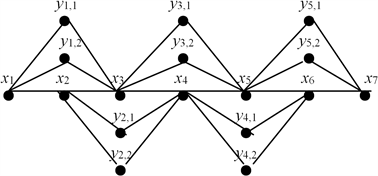Figure 1. P7(2)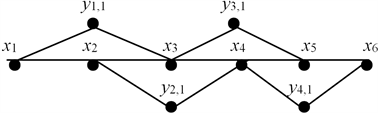Figure 2. P6(1)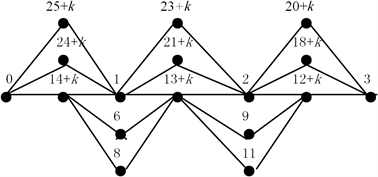Figure 3. P7(2) and k-graceful labelling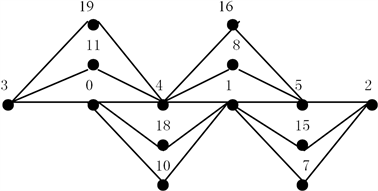Figure 4. P6(2) and sequential labelling

2. 定理的证明

$|V\left({P}_{n}\left(m\right)\right)|=mn-2m+n$$|E\left({P}_{n}\left(m\right)\right)|=2mn-4m+n-1$

2.1. 定理1的证明

$f\left({x}_{2i-1}\right)=i-1$, $i=\text{1},\cdots ,\left(n+\text{1}\right)/2$,

$f\left({x}_{2i}\right)=mn-3m+n-i+k-1$, $i=\text{1},\cdots ,\left(n-\text{1}\right)/2$,

$f\left({y}_{2i-1,j}\right)=2mn-2m+n-\left(2m-1\right)i-2j+k-1$, $i=\text{1},\cdots ,\left(n-\text{1}\right)/2$, $j=1,\cdots ,m$,

$f\left({y}_{2i,j}\right)=n-2m-2+\left(2m-1\right)i+2j$, $i=\text{1},\cdots ,\left(n-3\right)/2$, $j=1,\cdots ,m$ .

1) 不同的顶点其标号不同。

a) 显然有如下结论：

$f\left({x}_{2i-1}\right)\ne \text{}f\left({x}_{2j-1}\right)$ ( $i\ne j$, $1\le i,j\le \left(n+1\right)/2$ ),

$f\left({x}_{2i}\right)\ne \text{}f\left({x}_{2j}\right)$ ( $i\ne j$, $1\le i,j\le \left(n-1\right)/2$ ),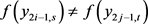( i = j 时, ； s = t 时, i ≠ j , 1 ≤ s , t ≤ m , 1 ≤ i , j ≤ ( n − 1 ) / 2 ),

$f\left({y}_{2i,s}\right)\ne \text{}f\left({y}_{2j,t}\right)$ ( $i=j$ 时, $s\ne t$$s=t$ 时, $i\ne j$, $\text{1}\le s,t\le m$, $1\le i,j\le \left(n-3\right)/2$ ).

b) 设

${\text{A}}_{0}=\left\{f\left({x}_{\text{2}i-\text{1}}\right)|\text{1}\le i\le \left(n+\text{1}\right)/2\right\}$, ${\text{C}}_{0}=\left\{f\left({y}_{2i-1,j}\right)|\text{1}\le i\le \left(n-\text{1}\right)/2,\text{1}\le j\le m\right\}$,

${\text{A}}_{\text{1}}=\left\{f\left({x}_{\text{2}i}\right)|\text{1}\le i\le \left(n-\text{1}\right)/2\right\}$, ${\text{C}}_{\text{1}}=\left\{f\left({y}_{2i,j}\right)|1\le i\le \left(n-3\right)/2,1\le j\le m\right\}$ .

${\text{A}}_{0}\cap {\text{A}}_{\text{1}}={\text{A}}_{0}\cap {\text{C}}_{0}={\text{A}}_{0}\cap {\text{C}}_{\text{1}}={\text{A}}_{\text{1}}\cap {\text{C}}_{0}={\text{A}}_{\text{1}}\cap {\text{C}}_{\text{1}}={\text{C}}_{0}\cap {\text{C}}_{\text{1}}=\varnothing$ .

$\mathrm{min}{\text{A}}_{0}=f\left({x}_{1}\right)=0$, $\mathrm{max}{\text{A}}_{0}=f\left({x}_{n}\right)=\left(n-1\right)/2$,

$\mathrm{min}{\text{A}}_{1}=f\left({x}_{n-}{}_{1}\right)=mn-3m+\left(n-1\right)/2+k$, $\mathrm{max}{\text{A}}_{1}=f\left({x}_{2}\right)=mn-3m+n-2+k$,

$\mathrm{min}{\text{C}}_{0}=f\left({y}_{n-2,m}\right)=mn-3m+3\left(n-1\right)/2+k$, $\mathrm{max}{\text{C}}_{0}=f\left({y}_{1,1}\right)=2mn-4m+n-2+k$,

$\mathrm{min}{\text{C}}_{1}=f\left({y}_{1,1}\right)=n-1$, $\mathrm{max}{\text{C}}_{1}=f\left({y}_{n-3,m}\right)=mn-3m+\left(n-1\right)/2$ .

2) $\mathrm{max}\left\{f\left(v\right)|v\in V\left({P}_{n}\left(m\right)\right)\right\}=\mathrm{max}{\text{C}}_{0}=2mn-4m+n-2+k=k+|E\left({P}_{n}\left(m\right)\right)|-1$

3) 不同的边其标号不同。

$\begin{array}{c}{\text{I}}_{1}=\left\{|f\left({y}_{2i-1,j}\right)-f\left({x}_{2i-1}\right)|,|f\left({y}_{2i-1,j}\right)-f\left({x}_{2i+1}\right)||1\le i\le \left(n-1\right)/2,1\le j\le m\right\}\\ =\left\{2mn-4m+n-2+k,2mn-4m+n-3+k,\cdots ,mn-3m+n-1+k\right\}\end{array}$,

$\begin{array}{c}{\text{I}}_{2}=\left\{|f\left({x}_{2i}\right)-f\left({x}_{2i-1}\right)|,|f\left({x}_{2i}\right)-f\left({x}_{2i+1}\right)||1\le i\le \left(n-1\right)/2\right\}\\ =\left\{mn-3m+n-2+k,mn-3m+n-3+k,\cdots ,mn-3m+k\right\}\end{array}$,

$\begin{array}{c}{\text{I}}_{3}=\left\{|f\left({y}_{2i,j}\right)-f\left({x}_{2i}\right)|,|f\left({y}_{2i,j}\right)-f\left({x}_{2i+2}\right)||1\le i\le \left(n-3\right)/2,1\le j\le m\right\}\\ =\left\{mn-3m-1+k,mn-3m-2+k,\cdots ,k\right\}\end{array}$ .

$\mathrm{max}{\text{I}}_{1}=2mn-4m+n-2+k$, $\mathrm{min}{\text{I}}_{1}=mn-3m+n-1+k$,

$\mathrm{max}{\text{I}}_{2}=mn-3m+n-2+k$, $\mathrm{min}{\text{I}}_{2}=mn-3m+k$,

$\mathrm{max}{\text{I}}_{3}=mn-3m-1+k$, $\mathrm{min}{\text{I}}_{3}=k$ .

$\mathrm{min}{\text{I}}_{3}<\mathrm{max}{\text{I}}_{3}<\mathrm{min}{\text{I}}_{2}<\mathrm{max}{\text{I}}_{2}<\mathrm{min}{\text{I}}_{1}<\mathrm{max}{\text{I}}_{1}$，进而有， ${\text{I}}_{i}\cap {\text{I}}_{j}=\varnothing$$i\ne j$$\text{1}\le i,j\le \text{3}$ 。于是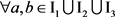，有 $a\ne b$

4) $\mathrm{max}\left\{{f}^{\prime }\left(e\right)|e\in E\left({P}_{n}\left(m\right)\right)\right\}=\mathrm{max}{\text{I}}_{1}=2mn-4m+n-2+k=k+|E\left({P}_{n}\left(m\right)\right)|-1$

$f\left({x}_{2i-1}\right)=i-1$, $i=\text{1},\cdots ,n/2$,

$f\left({x}_{2i}\right)=mn-2m+n-i+k-1$, $i=\text{1},\cdots ,n/2$,

$f\left({y}_{2i-1,j}\right)=2mn-2m+n-\left(2m-1\right)i-2j+k-1$, $i=1,\cdots ,\left(n-2\right)/2$, $j=1,\cdots ,m$,

$f\left({y}_{2i,j}\right)=n-2m-2+\left(2m-1\right)i+2j$, $i=1,\cdots ,\left(n-2\right)/2$, $j=1,\cdots ,m$ .

2.2. 定理2的证明

$\theta \left({x}_{2i-1}\right)=\left(n-1\right)/2+i$, $i=\text{1},\cdots ,\left(n+\text{1}\right)/2$,

$\theta \left({x}_{2i}\right)=i-1$, $i=\text{1},\cdots ,\left(n-\text{1}\right)/2$,

$\theta \left({y}_{2i-1,j}\right)=2mn-4m+3n-4-3i-2j\left(n-2\right)$, $i=\text{1},\cdots ,\left(n-\text{1}\right)/2$, $j=1,\cdots ,m$,

$\theta \left({y}_{2i,j}\right)=2mn-4m+5\left(n-1\right)/2-3i-2j\left(n-2\right)$, $i=\text{1},\cdots ,\left(n-\text{3}\right)/2$, $j=1,\cdots ,m$ .

1) 不同的顶点其标号不同。

a) 设

$\text{B}=\left\{\theta \left({x}_{\text{2}i}\right)|\text{1}\le i\le \left(n-1\right)/2\right\}\cup \left\{\theta \left({x}_{\text{2}i-\text{1}}\right)|\text{1}\le i\le \left(n+1\right)/2\right\}$,

${\text{C}}_{j}=\left\{\theta \left({y}_{2i-1,j}\right)|1\le i\le \left(n-1\right)/2\right\}$, $j=1,\cdots ,m$,

${\text{D}}_{j}=\left\{\theta \left({y}_{2i,j}\right)|1\le i\le \left(n-3\right)/2\right\}$, $j=1,\cdots ,m$ .

$\mathrm{min}\text{B}=0$, $\mathrm{max}\text{B}=n$,

$\mathrm{min}{\text{C}}_{j}=2mn-4m+\left(3n-5\right)/2-2j\left(n-2\right)$, $j=1,\cdots ,m$,

$\mathrm{max}{\text{C}}_{j}=2mn-4m+3n-7-2j\left(n-2\right)$, $j=1,\cdots ,m$,

$\mathrm{min}{\text{D}}_{j}=2mn-4m+n+2$, $j=1,\cdots ,m$,

$\mathrm{max}{\text{D}}_{j}=2mn-4m+5\left(n-1\right)/2-3-2j\left(n-2\right)$, $j=1,\cdots ,m$,

$\begin{array}{l}\mathrm{min}\text{B}<\mathrm{max}\text{B}<\mathrm{min}{\text{D}}_{m}<\mathrm{max}{\text{D}}_{m}<\mathrm{min}{\text{C}}_{m}<\mathrm{max}{\text{C}}_{m}<\mathrm{min}{\text{D}}_{m-}{}_{1}<\mathrm{max}{\text{D}}_{m-}{}_{1}\\ <\cdots <\mathrm{min}{\text{D}}_{1}<\mathrm{max}{\text{D}}_{1}<\mathrm{min}{\text{C}}_{1}<\mathrm{max}{\text{C}}_{1}\end{array}$ 。于是有 $\text{B}\cap {\text{C}}_{j}=\text{B}\cap {\text{D}}_{j}={\text{C}}_{j}\cap {\text{D}}_{j}=\varnothing$$j=1,\cdots ,m$ 。得 $\forall u,v\in V\left({P}_{n}\left(m\right)\right)$，若 $u\ne v$，有 $\theta \left(u\right)\ne \theta \left(v\right)$

2) $\mathrm{max}\left\{\theta \left(v\right)|v\in V\left({P}_{n}\left(m\right)\right)\right\}=2mn-4m+n-3<2mn-4m+n-1=|E\left({P}_{n}\left(m\right)\right)|$

3) 不同的边其标号不同。

${\text{J}}_{0}=\left\{\theta \left({x}_{i}\right)+\theta \left({x}_{i+}{}_{1}\right)|1\le i\le n-1\right\}=\left\{\left(n+1\right)/2,\left(n+1\right)/2+1,\cdots ,\left(n+1\right)/2+n-2\right\}$,

$\begin{array}{l}{\text{J}}_{1,j}=\left\{\theta \left({y}_{2i-1,j}\right)+\theta \left({x}_{2i+1}\right),\theta \left({y}_{2i-1,j}\right)+\theta \left({x}_{2i-1}\right)|1\le i\le \left(n-1\right)/2\right\}\\ \text{\hspace{0.17em}}\text{\hspace{0.17em}}\text{\hspace{0.17em}}\text{\hspace{0.17em}}\text{\hspace{0.17em}}=\left\{2mn-4m+\left(7n-11\right)/2-2j\left(n-2\right),2mn-4m+\left(7n-11\right)/2-1-2j\left(n-2\right),\\ \text{\hspace{0.17em}}\text{\hspace{0.17em}}\text{\hspace{0.17em}}\text{\hspace{0.17em}}\text{\hspace{0.17em}}\text{\hspace{0.17em}}\text{\hspace{0.17em}}\text{\hspace{0.17em}}\text{\hspace{0.17em}}\cdots ,2mn-4m+\left(5n-7\right)/2-2j\left(n-2\right)\right\},\text{\hspace{0.17em}}\text{\hspace{0.17em}}\text{\hspace{0.17em}}j=1,\cdots ,m\end{array}$,

$\begin{array}{c}{\text{J}}_{2,j}=\left\{\theta \left({y}_{\text{2}i,j}\right)+\theta \left({x}_{\text{2}i+\text{2}}\right)\text{},\theta \left({y}_{\text{2}i,j}\right)+\theta \left({x}_{\text{2}i}\right)|\text{1}\le i\le \left(n-\text{3}\right)/2\right\}\\ =\left\{2mn-4m+\left(5n-9\right)/2-2j\left(n-2\right),\cdots ,2mn-4m+\left(3n-1\right)/2-2j\left(n-2\right)\right\},\text{\hspace{0.17em}}\text{\hspace{0.17em}}j=1,\cdots ,m\end{array}$,

$\begin{array}{c}{\text{J}}_{\text{1}}={\text{J}}_{\text{2},m}\cup {\text{J}}_{\text{1},m}\\ =\left\{\left(3n-1\right)/2,\left(3n-1\right)/2+1,\cdots ,\left(5n-9\right)/2\right\}\\ \text{\hspace{0.17em}}\text{\hspace{0.17em}}\text{ }\text{ }\cup \left\{\left(5n-7\right)/2,\left(5n-7\right)/2+1,\cdots ,\left(7n-11\right)/2\right\}\end{array}$,

$\begin{array}{c}{\text{J}}_{\text{2}}={\text{J}}_{\text{2},m-\text{1}}\cup {\text{J}}_{\text{1},m-\text{1}}\\ =\left\{\left(7n-9\right)/2,\left(7n-9\right)/2+1,\cdots ,\left(9n-17\right)/2\right\}\\ \text{\hspace{0.17em}}\text{\hspace{0.17em}}\text{\hspace{0.17em}}\cup \left\{\left(9n-15\right)/2,\left(9n-15\right)/2+1,\cdots ,\left(11n-19\right)/2\right\}\end{array}$,

$\begin{array}{c}{\text{J}}_{m}={\text{J}}_{\text{2},\text{1}}\cup {\text{J}}_{\text{1},\text{1}}\\ =\left\{2mn-4m-\left(n-7\right)/2,2mn-4m-\left(n-7\right)/2+1,\cdots ,2mn-4m+\left(n-1\right)/2\right\}\\ \text{\hspace{0.17em}}\text{\hspace{0.17em}}\text{ }\text{ }\cup \left\{2mn-4m+\left(n+1\right)/2,2mn-4m+\left(n+1\right)/2+1,\cdots ,2mn\text{}-4m+3\left(n-1\right)/2\right\}\end{array}$

4) $\begin{array}{c}\text{J}={\text{J}}_{0}\cup {\text{J}}_{\text{1}}\cup {\text{J}}_{\text{2}}\cup \cdots \cup {\text{J}}_{m}\\ =\left\{\left(n+1\right)/2,\left(n+1\right)/2+1,\cdots ,2mn-4m+3\left(n-1\right)/2\right\}\\ =\left\{\left(n+1\right)/2,\left(n+1\right)/2+1,\cdots ,\left(n+1\right)/2+|E\left({P}_{n}\left(m\right)\right)|\right\}\end{array}$

$\theta \left({x}_{2i-1}\right)=n/2+i$, $i=1,\cdots ,n/2$,

$\theta \left({x}_{2i}\right)=i-1$, $i=1,\cdots ,n/2$,

$\theta \left({y}_{2i-1,j}\right)=2mn-4m+3n-4-3i-2j\left(n-2\right)$, $i=1,\cdots ,\left(n-2\right)/2$, $j=1,\cdots ,m$,

$\theta \left({y}_{2i,j}\right)=2mn-4m+\left(5n-2\right)/2-3i-2j\left(n-2\right)$, $i=1,\cdots ,\left(n-2\right)/2$, $j=1,\cdots ,m$ .

$\theta \left({x}_{2i-1}\right)=\left(n-3\right)/2+i$, $i=1,\cdots ,\left(n+1\right)/2$,

$\theta \left({x}_{2i}\right)=i-1$, $i=1,\cdots ,\left(n-1\right)/2$,

$\theta \left({y}_{2i-1,j}\right)=2mn-4m+3n-4-3i-2j\left(n-2\right)$, $i=1,\cdots ,\left(n-1\right)/2$, $j=1,\cdots ,m$,

$\theta \left({y}_{2i,j}\right)=2mn-4m+\left(5n-7\right)/2-3i-2j\left(n-2\right)$, $i=1,\cdots ,\left(n-3\right)/2$, $j=1,\cdots ,m$ .

$\theta \left({x}_{2i-1}\right)=\left(n-2\right)/2+i$, $i=1,\cdots ,n/2$,

$\theta \left({x}_{2i}\right)=i-1$, $i=1,\cdots ,n/2$,

$\theta \left({y}_{2i-1,j}\right)=2mn-4m+3n-4-3i-2j\left(n-2\right)$, $i=1,\cdots ,\left(n-2\right)/2$, $j=1,\cdots ,m$,

$\theta \left({y}_{2i,j}\right)=2mn-4m+\left(5n-4\right)/2-3i-2j\left(n-2\right)$, $i=1,\cdots ,\left(n-2\right)/2$, $j=1,\cdots ,m$ .

  马克杰. 优美图[M]. 北京: 北京大学出版社, 1991.  路线, 李秀芬. 关于链路Pn2*的优美性[J]. 上侥师专学报, 1994, 6(14): 26-30.